### GATE 2008 ECE Video Solutions on Analog Circuits (Analog Electronics)

1. Consider the following circuit using an ideal OP-AMP. The I-V characteristic of the diode is described by the relation I = Io(exp(V/VT) - 1), where VT = 25 mV, Io = 1 µA and V is the voltage across the diode(taken as positive for forward bias). For an input voltage Vi = - 1 volts, the output voltage Vo isa. 0 volts
b. 0.1 volts
c. 0.7 volts
d. 1.1 volts

2. The OP-AMP circuit shown below represents aa. High pass filter
b. Low pass filter
c. Band pass filter
d. Band reject filter

3. Consider the schmitt trigger circuit shown below.A triangular wave which goes from –12 volts to +12 volts is applied to the inverting input of OP-AMP. Assume that the output of the OP-AMP swings from +15 volts to –15 volts. The voltage at the non-inverting input switches between
a. – 12 volts and +12 volts
b. – 7.5 volts and +7.5 volts
c. – 5 volts and +5 volts
d. 0 volts and +5 volts

In the following transistor circuit, VBE = 0.7 volts, re = 25 mV/IE, β and all the capacitances are very large.4. The value of DC current IE is
a. 1 mA
b. 2 mA
c. 5 mA
d. 10 mA

5. The mid-band voltage gain of the amplifier is approximately
a. – 180
b. – 120
c. – 90
d. – 60

### GATE 2009 ECE Video Solutions on Analog Circuits (Analog Electronics)

1. In the following Astable Multivibrator circuit, which properties of Vo(t) depend on R2 ?a. Only the frequency
b. Only the amplitude
c. Both the amplitude and the frequency
d. Neither the amplitude nor the frequency

2. In the circuit shown below, the OP-AMP is ideal, the transistor has VBE = 0.6 volts and β = 150. Decide whether the feedback in the circuit is positive or negative and determine the voltage V at the output of the OP-AMP.a. Positive feedback, V = 10 Volts
b. Positive feedback, V = 0 Volts
c. Negative feedback, V = 5 Volts
d. Negative feedback, V = 2 Volts

3. A small signal source Vi(t) = Acos20t + Bsin106t is applied to a transistor amplifier as shown below. The transistor has β = 150 and hie = 3 KΩ. Which expression best approximate Vo(t).a. Vo(t) = - 1500 (Acos20t + Bsin106t)
b. Vo(t) = - 150 (Acos20t + Bsin106t)
c. Vo(t) = - 1500 (Bsin106t)
d. Vo(t) = - 150 (Bsin106t)

### GATE 2010 ECE Video Solutions on Analog Circuits (Analog Electronics)

1. The amplifier circuit shown below uses a silicon transistor. The capacitors CC and CE can be assumed to be short at signal frequency and effect of output resistance ro can be ignored. If CE is disconnected from the circuit, which one of the following statements is TRUE.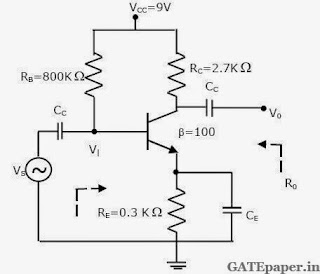a. The input resistance Ri increases and magnitude of voltage gain AV decreases
b. The input resistance Ri decreases and magnitude of voltage gain AV increases
c. The input resistance Ri decreases and magnitude of voltage gain AV decreases
d. The input resistance Ri increases and magnitude of voltage gain AV increases

2. In the silicon BJT circuit shown below, assume that the emitter area of transistor Q1 is half that of transistor Q2. The value of current Io is approximatelya. 0.5 mA
b. 2 mA
c. 9.3 mA
d. 15 mA

3. Assuming the OP-AMP is ideal, the voltage gain of the amplifier shown below isa. - R2/R1
b. - R3/R1
c. - (R2 || R3) / R1
d. - (R2 + R3)/R1

4. The transfer characteristic for the precision rectifier circuit shown below is (Assume ideal OP-AMP and Practical Diodes)Common Data Questions:
Consider the common emitter amplifier shown below with the following circuit parameters.
β = 100, gm = 0.3861 A/V, ro = 259 Ω, RS = 1 KΩ, RB = 93 KΩ, RC = 250 Ω, RL = 1 KΩ, C1 = ∞ and C2 = 4.7 µF.5. The resistance seen by the source VS is
a. 258 Ω
b. 1258 Ω
c. 93 KΩ
d. ∞

6. The lower cutoff frequency due to C2 is
a. 33.9 Hz
b. 27.1 Hz
c. 13.6 Hz
d. 16.9 Hz

### GATE 2011 ECE Video Solutions on Analog Circuits (Analog Electronics)

1. In the circuit shown below, capacitors C1 and C2 are very large and are shorts at the input frequency . vi is a small signal input. The gain magnitude |V0/Vi| at 10 M rad/sec is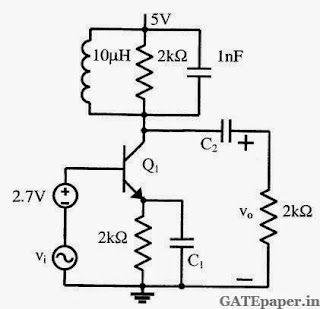a. Maximum
b. Minimum
c. Unity
d. Zero

2. The circuit below implements a filter between the input current ii and the output voltage vo. Assume that the op-amp is ideal. The filter implemented is aa. Low pass filter
b. Band pass filter
c. Band stop filter
d. High pass filter

In the circuit shown below, assume that the voltage drop across a forward biased diode is 0.7 votls. The thermal voltage VT = KT/q = 25 mV. The small signal input vi = 100 cos(ωt) mV.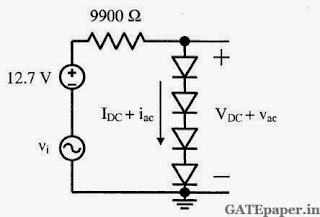3. The bias current IDC through the diodes is
a. 1 mA
b. 1.28 mA
c. 1.5 mA
d. 2 mA

4. The ac output voltage VAC is
a. 0.25 cos(ωt) mV
b. 1 cos(ωt) mV
c. 2 cos(ωt) mV
d. 22 cos(ωt) mV

### GATE 2012 ECE Video Solutions on Analog Circuits (Analog Electronics)

1. The current ib through the base of a silicon NPN transistor is 1+0.1cos (10000πt) mA. At 300oK, the rπ in the small signal model of the transistor is ________ (in Ohms).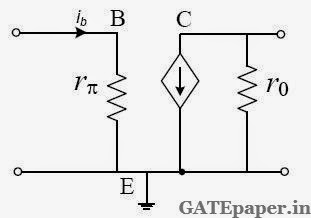a. 250
b. 27.5
c. 25
d. 22.5

2. The impedance looking into nodes 1 and 2 in the given circuit is________ (in Ohms).a. 50
b. 100
c. 5K
d. 10.1 K

3. The circuit shown is a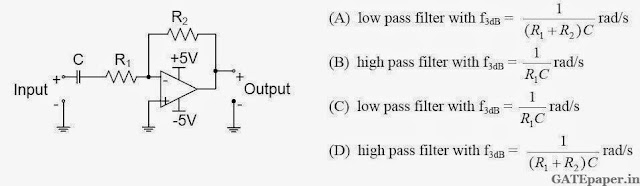4. The voltage gain Av, of the circuit shown below isa. |Av| ≡ 200
b. |Av| ≡ 100
c. |Av| ≡ 20
d. |Av| ≡ 10